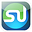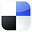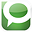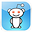# Using the Hypothesis Test in Excel To TestHeadlines

This article will show exactly how to perform a Hypothesis Test in Excel to test whether one headline performs better than another headline in a pay-per-click Internet marketing campaign. This Hypothesis Test will be testing the Null Hypothesis that both headlines perform at the same level.

Specifically, we will show how to use Excel to perform a One-Tailed test, a Two-Sample test , Unpaired, Hypothesis Test of Proportion to determine whether Headline 2 converts better than Headline 1 when used in the same PPC ad. Different types of Hypothesis Tests such as a Paired test, a Two-tailed test, and a Hypothesis Test of Mean are demonstrated in other articles of this blog.

The advantages of statistical analysis in Excel to solve business statistics problems is that most problems can be solved in just one or two steps and there is no more need to look anything up on Normal Distribution tables.

Here is the problem:

Problem: An Internet marketing manager is testing the effectiveness of two different headlines when used in the same pay-per-click ad. The Internet marketing manager is trying to determine whether Headline 2 is better than Headline 1. Headline 1 was inserted into a PPC ad. Running this Headline 1/ad combination resulted in 80 click-throughs. 52 of those 80 click-through visitors converted (purchased).

Headline 2 was run with the same PPC ad under the same conditions and approximately the same number of ad impressions. Running this combination of Headline 2/ad resulted in 90 click-throughs, of which 63 converted. Determine within an error of 1% whether Headline 2 converted better than Headline 1.

Here are the Conversion Data for Headlines 1 and  2:

p1avg = Sample proportion 1 = 52 / 80 = 0.65 converted

q1avg = 1 - p1avg = 1 - 0.65 = 0.35 not converted

n1 = Sample size 1 = 80

p2avg = Sample proportion 2 = 63 / 90 = 0.70 converted

q2avg = 1 - p2avg = 1 - 0.70 = 0.30 not cconverted

n2 = Sample size 2 = 90

α = 0.01 = alpha = Level of significance, therefore there is a 1% max chance of error.

This results in a 99% Level of Certainty Required

Before we begin solving this problem, we need to know whether we are dealing with normally distributed data. If the data is not normally distributed, we have to use nonparametric statistical tests to solve this problem.

Always Test for Normality First

Normality tests should be performed on both Headline 1 and Headline 2 conversion data. Both data sets must be normally distributed to perform the well-known hypothesis test that is based upon the underlying data being normally distributed. This blog has numerous articles about how to perform normality testing and nonparametric testing if the data is not normally distributed.

The MOST Important Step

Determine What Type of Hypothesis

Test You Will Perform﻿

1) Hypothesis Test of Mean or Proportion?

We know that this is a test of proportion and not mean because because each individual sample taken has only 2 possible values: the headline/ad combination either converted the click-through visitor or it didn't.

2) One or Two-Tailed Hypothesis Test?

We know that this is a one-tailed test because we are trying to determine if Headline 2 has a higher conversion rate than Headline 1, not whether conversion rates are merely different, which would be a two-tailed test.

3) One or Two-Sample Hypothesis Test?

We know that two samples must to be taken because no data is initially available.

4) Paired or Unpaired Hypothesis Test?

This is unpaired data because groups are sampled independently. Hypothesis tests of proportion cannot be applied to paired data.

In this case, we are performing a One-Tailed, Two-Sample, Unpaired Hypothesis Test of Proportion to determine whether Headline 2 has a higher conversion rate than Headline 1. We will do this test in Excel. It is extremely important to establish the type of Hypothesis test.

Each type of Hypothesis test uses a slightly (or very) different methodology and set of formulas. The previous 2 articles in this blog with provide examples of Hypothesis Tests of Mean. You will notice that the formulas for Mean Hypothesis Tests are completely different, but the 4-step method always works for any type of Hypothesis Test.

The Four-Step Method That Solves ALL Hypothesis Tests

This problem can be solved using the standard four-step method for Hypothesis testing.

Step 1 - Create the Null and Alternate Hypotheses

The Null Hypothesis normally states that both populations sampled are the same. If the proportions p1avg and p2avg, conversion rates from each headline are the same, then p1avg - p2avg = 0.

The Null Hypothesis states that both headlines the same conversion rates, which is equivalent to:

Null Hypothesis = H0 = p1avg - p2avg = 0

The Alternate Hypothesis states that Headline 2 is better than Headline 1, which is equivalent to:

The Alternate Hypothesis, which states that p2avg is greater than p1avg, is as follows:

Alternate Hypothesis, H1 = p1avg - p2avg is less than 0

****************************

For this one-tailed test, the Alternative Hypothesis states that if the value of the distributed variable (p1avg - p2avg) is less than the value stated by the Null Hypothesis, the Region of Uncertainty will be in the outer left tail.

Note - the Alternative Hypothesis determines whether the Hypothesis test is a one-tailed test or a two-tailed test as follows:

One-tailed test - (Value of variable) is greater than OR is less than (Constant)

Two-tailed test - (Value of variable) does not equal (Constant)

Step 2 - Map the Normal Curve

We now create a Normal curve showing a distribution of the same variable that is used by the Null Hypothesis, which is (p1avg - p2avg). The mean of this Normal curve will occur at the same value of the distributed variable as stated in the Null Hypothesis.

Since the Null Hypothesis states that p1avg - p2avg = 0, the Normal curve will map the distribution of the variable (p1avg - p2avg) with a mean of (p1avg - p2avg) = 0

This Normal curve will have a standard error that is calculated as the standard error of a sampled proportion is normally calculated, as follows:

The standard error of the difference of proportions is:

sp1avg-p2avg =

= SQRT [ pweighted * qweighted ( 1 / n1 + 1 / n2 ) ]

pweighted = (n1*p1avg + n2*p2avg) / (n1 + n2)

= (n1*p1avg + n2*p2avg) / (n1 + n2)

= [ (80 * 0.65) + (90 * 0.70) / (80 + 90) ]

= 0.676

qweighted = 1 - pweighted

= 1 - pweighted

= 1 - 0.676

= 0.324

Standard Error = sp1avg-p2avg =

= SQRT [ pweighted * qweighted ( 1 / n1 + 1 / n2 ) ]

= SQRT [ ( 0.676 * 0.324 ) * ( 1 / 80 + 1 / 90 ) ]

= 0.0719

Click On Image To See Larger Version

The problem requires a 99% Level of Certainty so the Region of Certainty will contain 99% of the area under the Normal curve.

We know that this problem uses a one-tailed test with the Region of Uncertainty entirely contained in the outer left tail.

The Region of Uncertainty contains 1% of the total area under the Normal curve. The entire 99% Region of Certainty lies to the right of the 1% Region of Uncertainty, which is entirely contained in the outer left tail.

****************************************

We need to find out how far the boundary of the Region of Certainty is from the Normal curve mean. Calculating the number of standard errors from the Normal curve mean to the outer boundary of the Region of Certainty in the right tail for a one-tailed test is done as follows:

z99%,1-tailed = NORMSINV(1 - α) = NORMSINV(0.99) = 2.33

Excel Note - NORMSINV(x) = The number of standard errors from the Normal curve mean to a point right of the Normal curve mean at which x percent of the area under the Normal curve will be to the left of that point.

Additional note - For a one-tailed test, NORMSINV(x) can be used to calculate the number of standard errors from the Normal curve mean to the boundary of the Region of Certainty whether it is in the left or the right tail.

The Region of Certainty extends to the left of the Normal curve mean of (p1avg - p2avg) = 0 by 2.33 standard errors.

One standard error = sp1avg-p2avg = 0.0719, so:
2.33 standard errors = (2.33) * (0.0719) = 0.1675

The outer left boundary of the Region of Certainty has the value
= µ - z99%,1-tailed * s(p1avg-p2avg)

which equals 0 - (2.33) * (0.0719) = - 0.1675

This point (-0.1675) is 2.33 standard errors to the left of the Normal curve mean of (p1avg - p2avg) = 0

This point (-0.1675) is the left boundary of the 99% Region of Certainty on the Normal curve.

Click Image To See Larger Version

Step 4 - Perform Critical Value and p-Value Tests

a) Critical Value Test

The Critical Value Test is the final test to determine whether to reject or not reject the Null Hypothesis. The p Value Test, described below, is an equivalent alternative to the Critical Value Test.

The Critical Value test tells whether the value of the actual variable, p1avg - p2avg, falls inside or outside of the Critical Value, which is the boundary between the Region of Certainty and the Region of Uncertainty.

If the actual value of the distributed variable, p1avg - p2avg, falls within the Region of Certainty, the Null Hypothesis is not rejected.

If the actual value of the distributed variable, p1avg - p2avg, falls outside of the Region of Certainty and, therefore, into the Region of Uncertainty, the Null Hypothesis is rejected and the Alternate Hypothesis is accepted.

In this case, the actual value of the variable, p1avg - p2avg =
= 0.65 - 070 = -0.05

The actual value of the variable (p1avg - p2avg) = -0.05 and is therefore inside the Critical Value (-0.1675), which is the boundary between the Regions of Certainty and Uncertainty.

The actual value of the variable (p1avg - p2avg) is inside the Region of Certainty and therefore inside the Critical Value.

We therefore do not reject the Null Hypothesis and state that the Null Hypothesis is not disproven that both headlines have the same conversion rates..

Click On Image To See Larger Version﻿

b) p Value Test

The p Value Test is an equivalent alternative to the Critical Value Test and also tells whether to reject or not reject the Null Hypothesis.

The p Value equals the percentage of area under the Normal curve that is in the tail outside of the actual value of the variable (p1avg - p2avg).

For a one-tailed test, if the p Value is larger than α, the Null Hypothesis is not rejected.

For a two-tailed test, if the p Value is larger than α/2, the Null Hypothesis is not rejected.

For a one-tailed test, the Region of Uncertainty is contained entirely in one tail. Therefore the curve area contained by the Region of Uncertainty in that tail equals α.

For a two-tailed test, the Region of Uncertainty is split between both tails. Therefore the curve area contained by the Region of Uncertainty in that tail equals α/2.

The p Value for the actual value of the distributed variable, which in this case is greater than the mean (falls to the left of the mean in the left tail), is:

p Value(p1avg-p2avg) =
= NORMSDIST( [ (p1avg - p2avg) - µ ] / s(p1avg - p2avg) )

**************************************

Excel note - NORMSDIST(x) calculates the total area under the Normal curve to the LEFT of the point that is x standard errors to the right of the Normal curve mean. If we are calculating the area to the RIGHT of this point, we would use 1 - NORMSDIST. This would be as follows:

p Value(p1avg-p2avg) =

= 1 - NORMSDIST( [ (p1avg - p2avg) - µ ] / s(p1avg - p2avg) )

******************************************************

Since we are calculating the area to the left of this point, we use:

p Value(p1avg-p2avg) =

= NORMSDIST( [ (p1avg - p2avg) - µ ] / s(p1avg - p2avg) )

= NORMSDIST((-0.05 - 0 ) / 0.0719) = NORMSDIST(-0.05/0.0719) = 0.24

The p Value (0.24) is greater than α (0.01), so the Null Hypothesis is not rejected.

For a one-tailed test- When the p Value is greater than α, the actual value of the distributed variable falls inside the Region of Certainty and the Null Hypothesis is not rejected.

This is the case here.

Click Image To See Larger Version

*****************************************

If You Like This, Then Share It...Excel Master Series Blog Directory

Statistical Topics and Articles In Each Topic

• Histograms in Excel
• Bar Chart in Excel
• Combinations & Permutations in Excel
• Normal Distribution in Excel
• t-Distribution in Excel
• Binomial Distribution in Excel
• z-Tests in Excel
• t-Tests in Excel
• Hypothesis Tests of Proportion in Excel
• Chi-Square Independence Tests in Excel
• Chi-Square Goodness-Of-Fit Tests in Excel
• F Tests in Excel
• Correlation in Excel
• Pearson Correlation in Excel
• Spearman Correlation in Excel
• Confidence Intervals in Excel
• Simple Linear Regression in Excel
• Multiple Linear Regression in Excel
• Logistic Regression in Excel
• Single-Factor ANOVA in Excel
• Two-Factor ANOVA With Replication in Excel
• Two-Factor ANOVA Without Replication in Excel
• Creating Interactive Graphs of Statistical Distributions in Excel
• Solving Problems With Other Distributions in Excel
• Optimization With Excel Solver
• Chi-Square Population Variance Test in Excel
• Analyzing Data With Pivot Tables
• SEO Functions in Excel
• Time Series Analysis in Excel